# R S Aggarwal Solutions for Class 11 Maths Chapter 26 Three Dimensional Geometry

R S Aggarwal Solutions Class 11 Maths Solutions Chapter 26 are provided here. Chapter 26 – Three Dimensional Geometry of RS Aggarwal Solutions includes three exercises and all questions are solved by BYJU’S subject experts for students to clear all their doubts on this topic. In this chapter, students will study about coordinates of a point in space, distance between two points, coordinates of the midpoint of a line segment and many more. RS Aggarwal Solutions for Class 11 Maths Chapter 26 includes solved problems and step by step explanations given by our experts to further help students to solve 3D geometric problems.

## Download PDF of R S Aggarwal Solutions for Class 11 Maths Chapter 26 Three Dimensional Geometry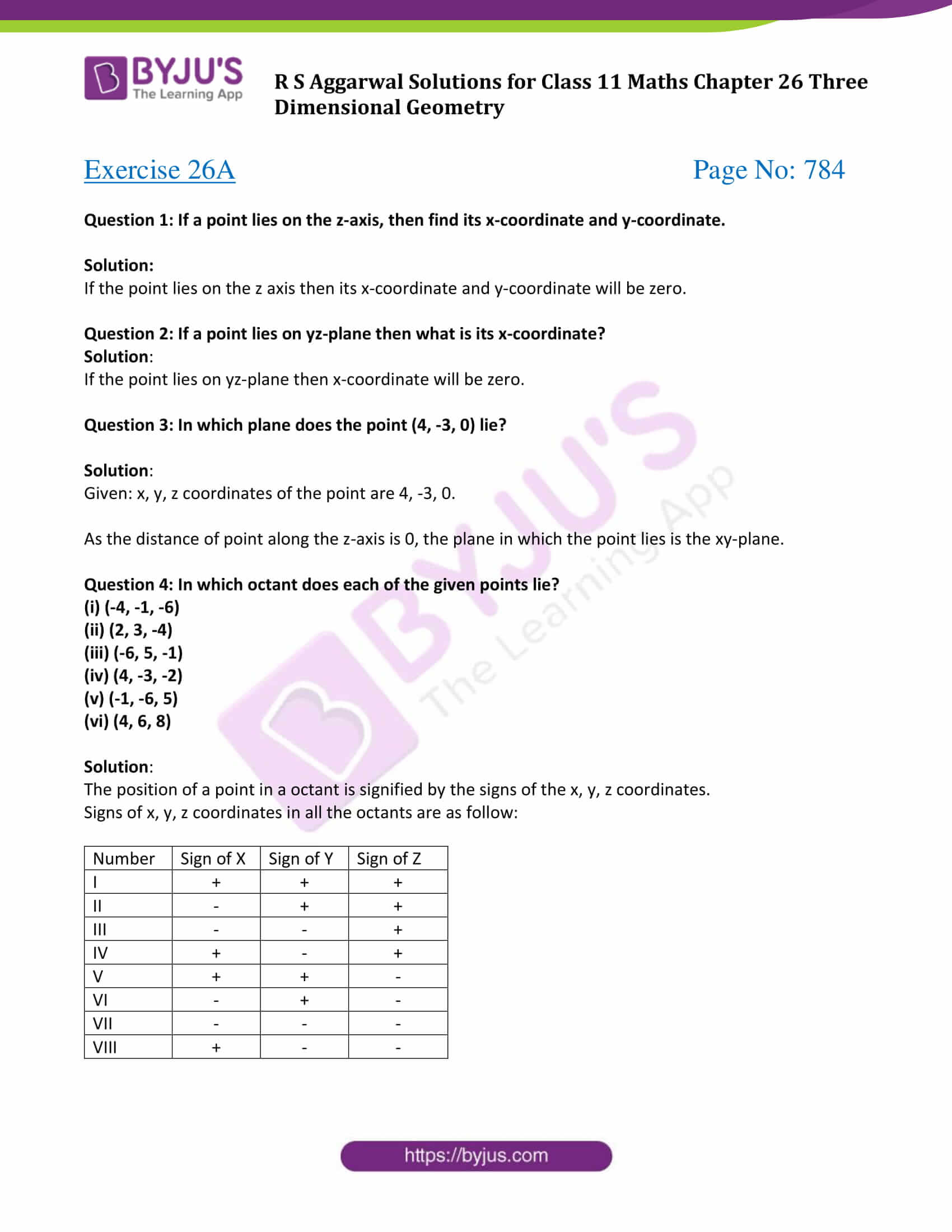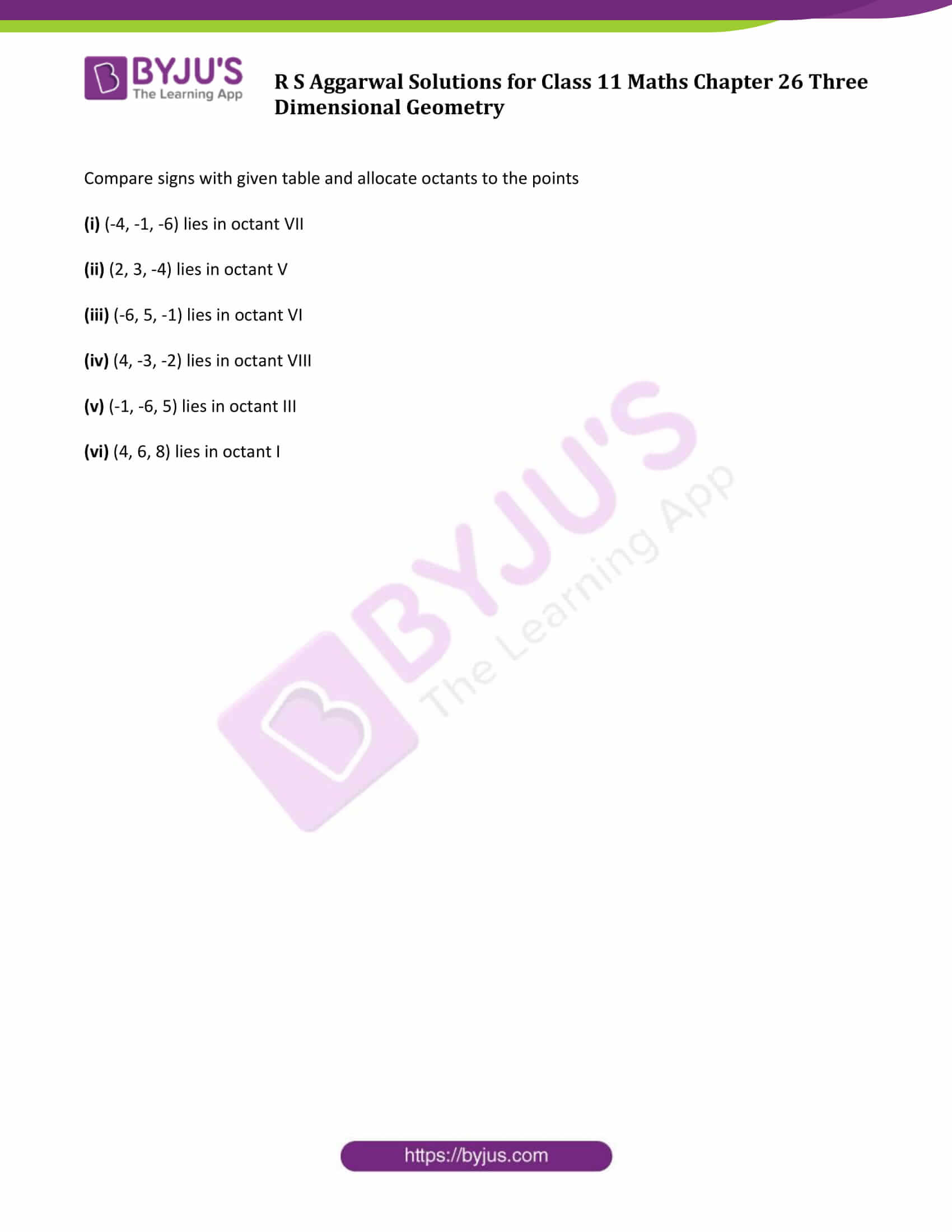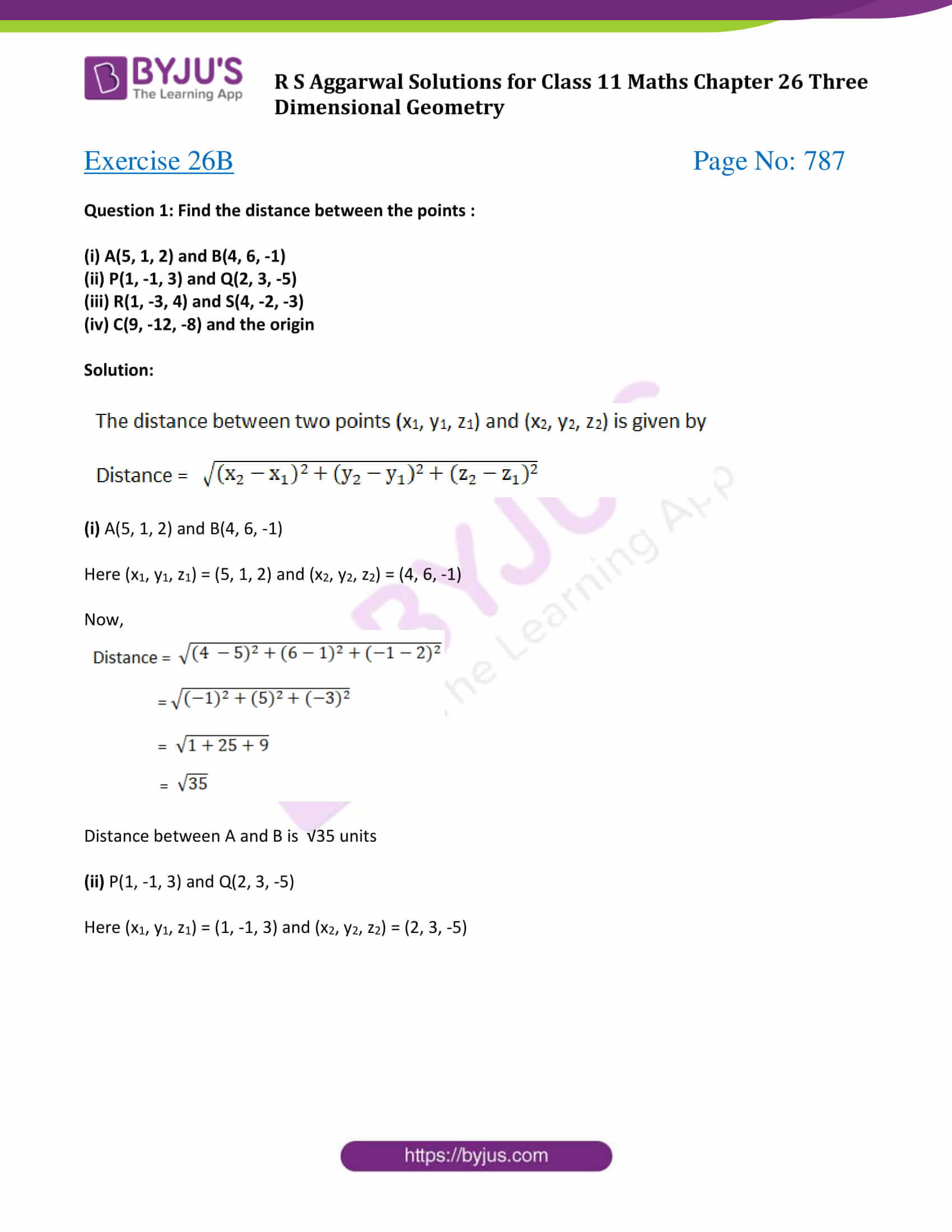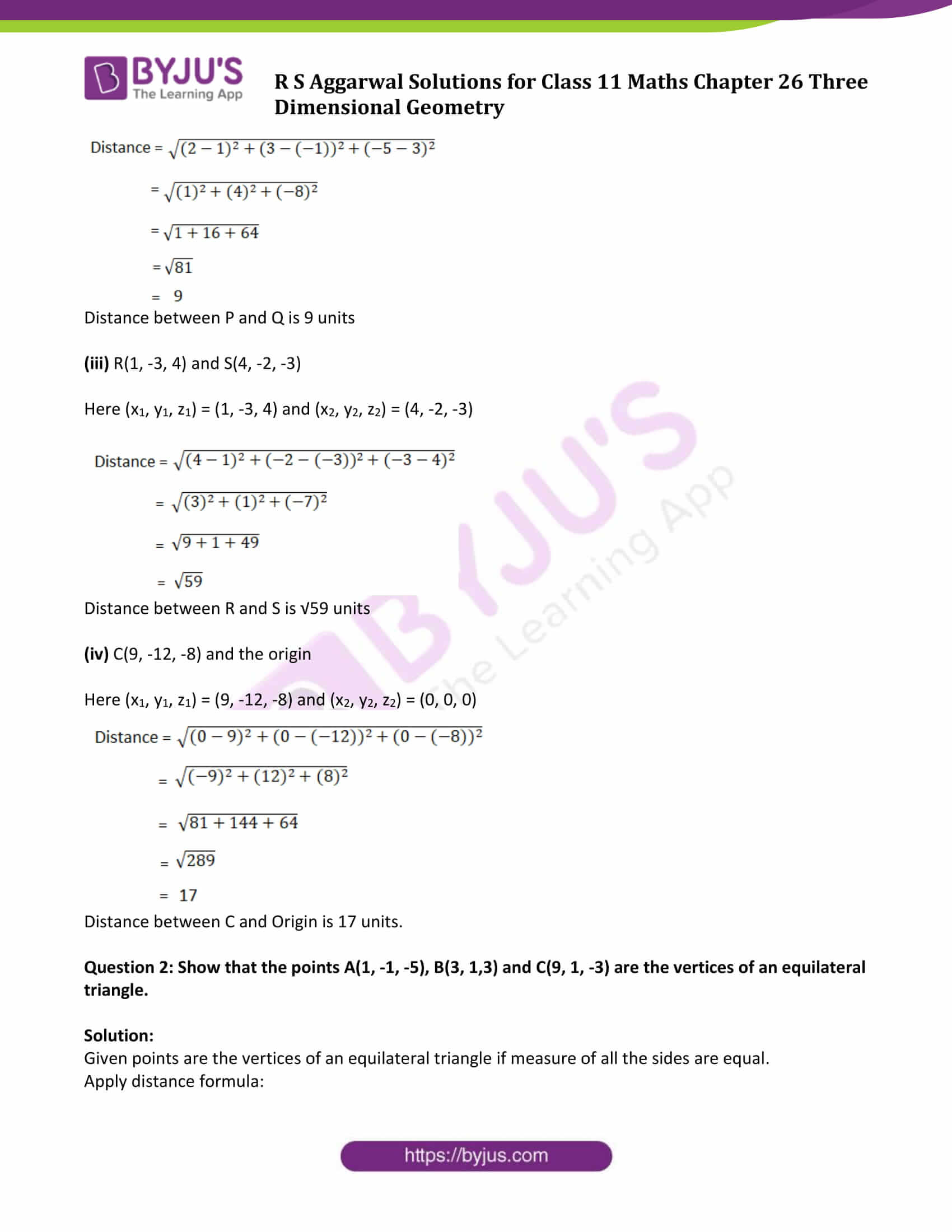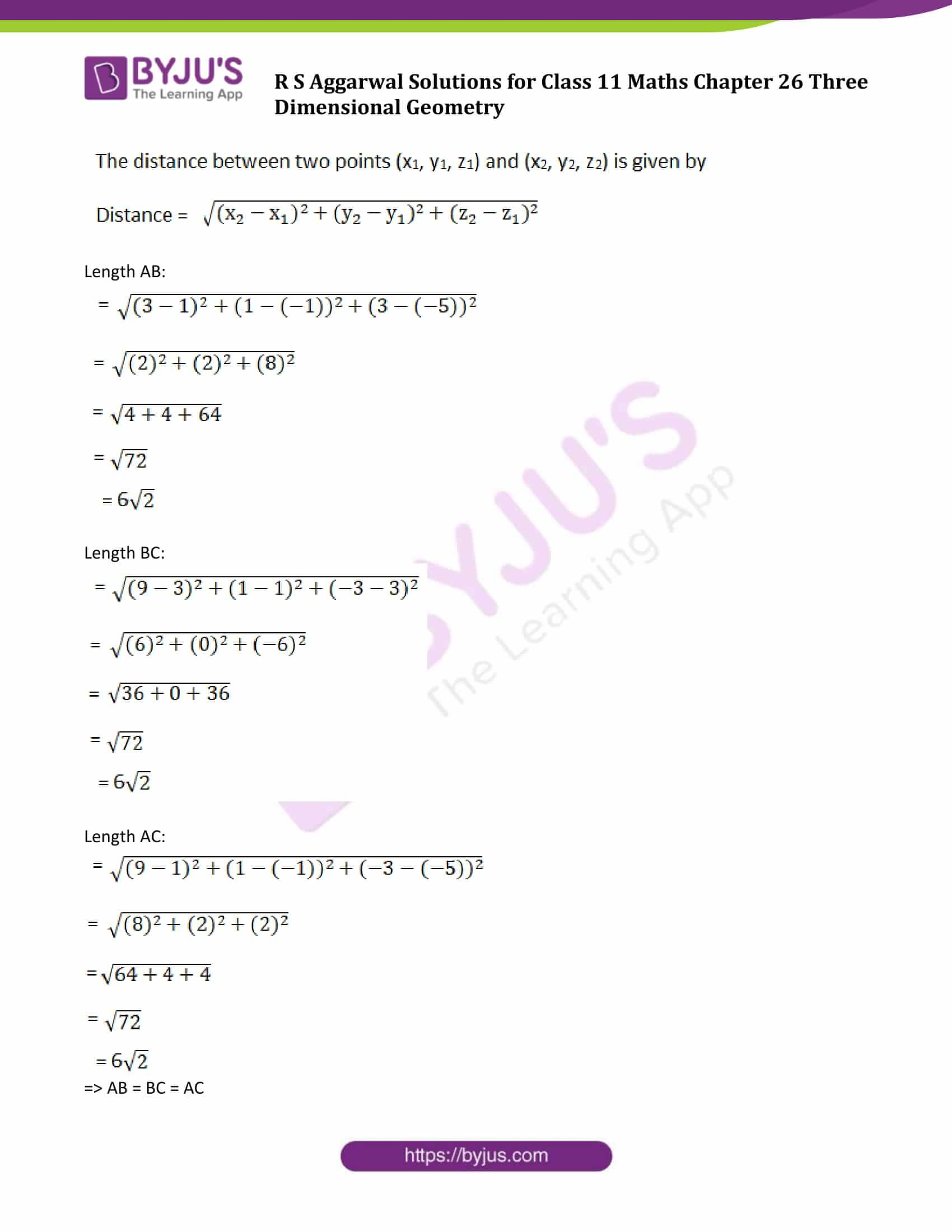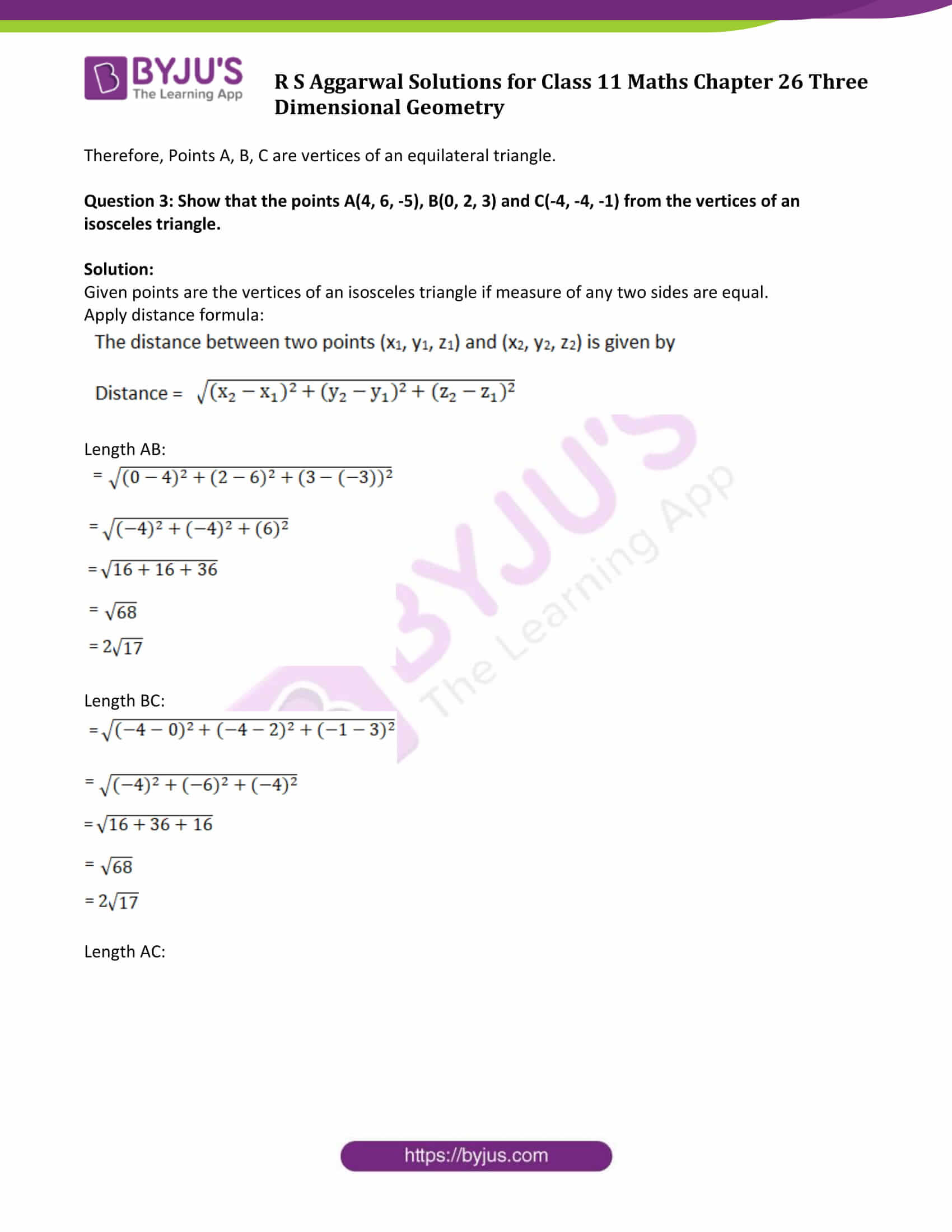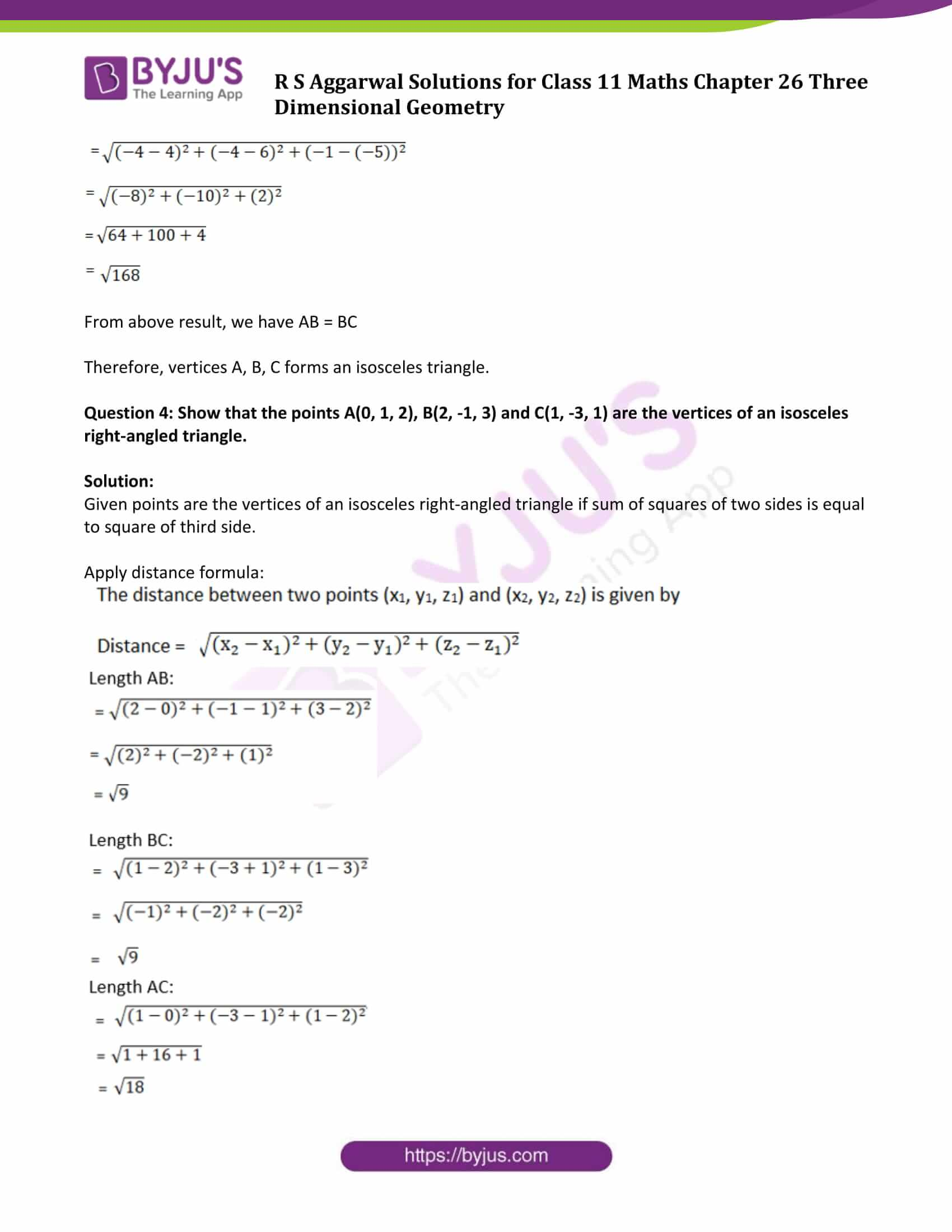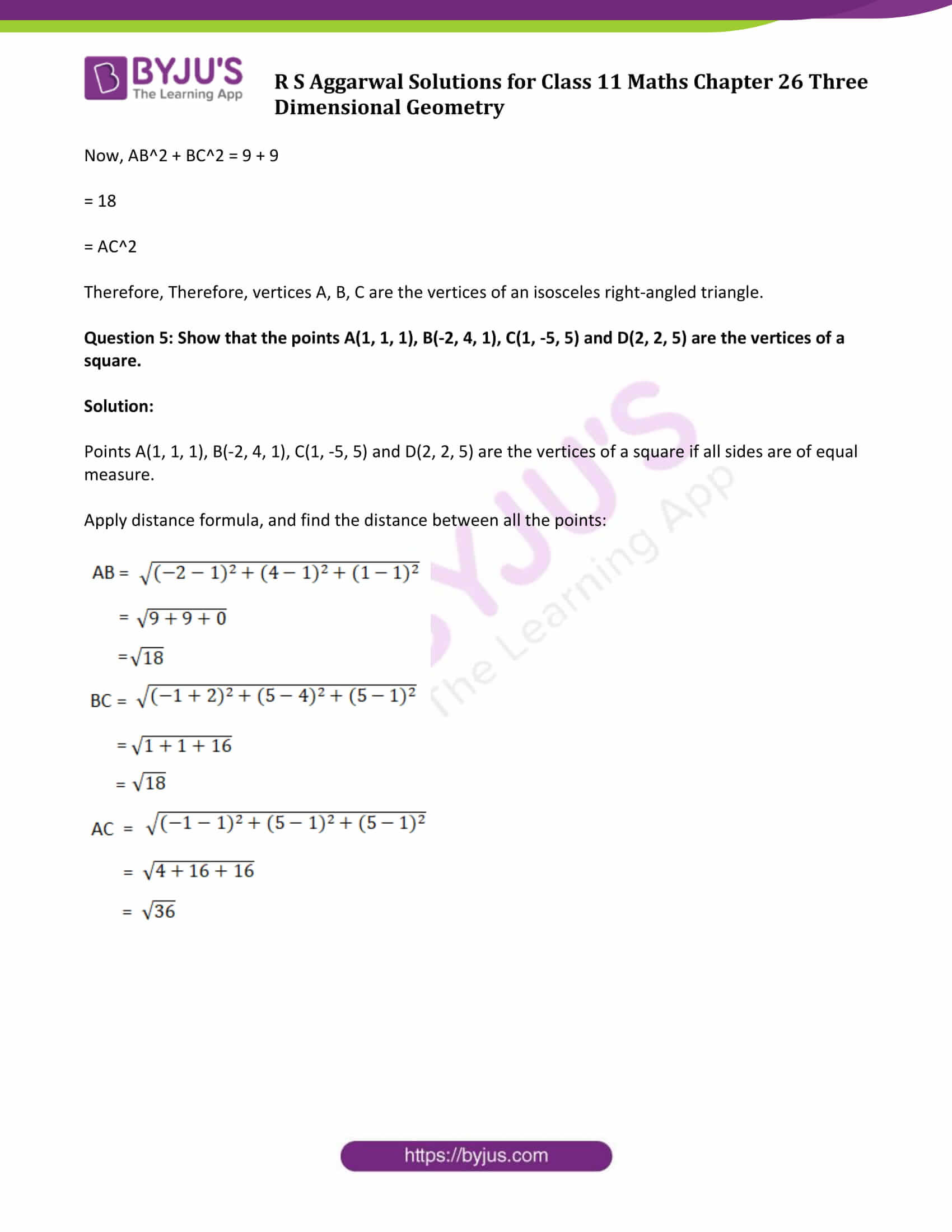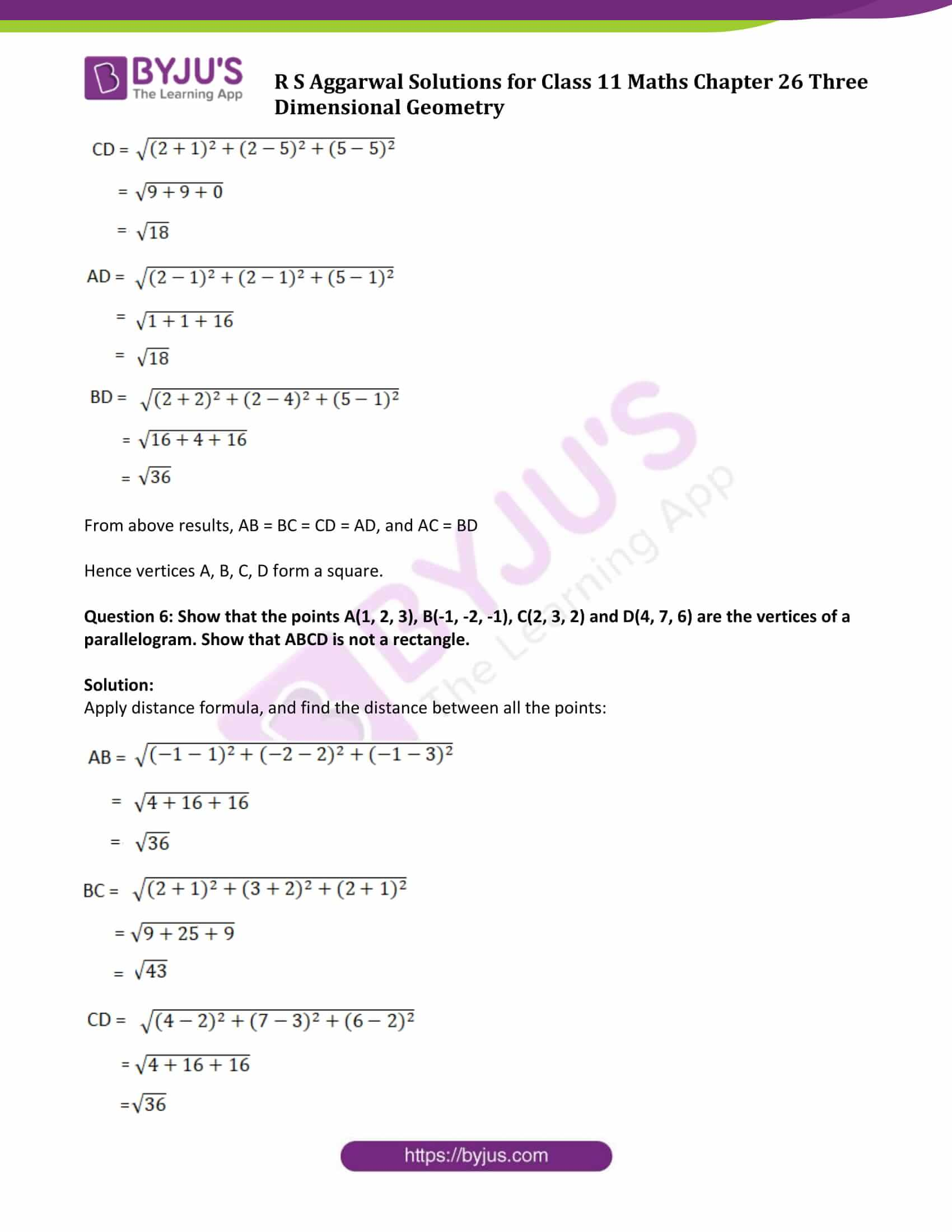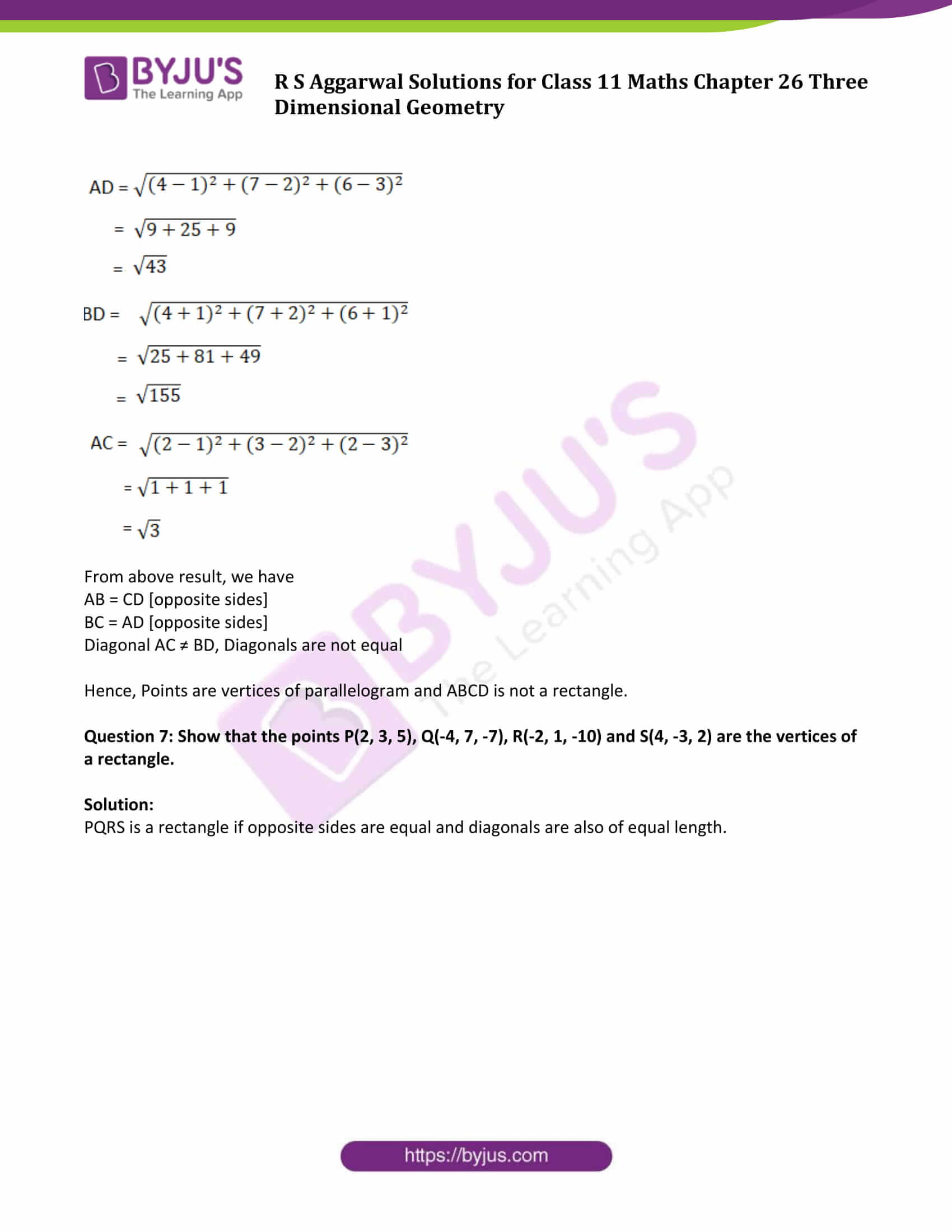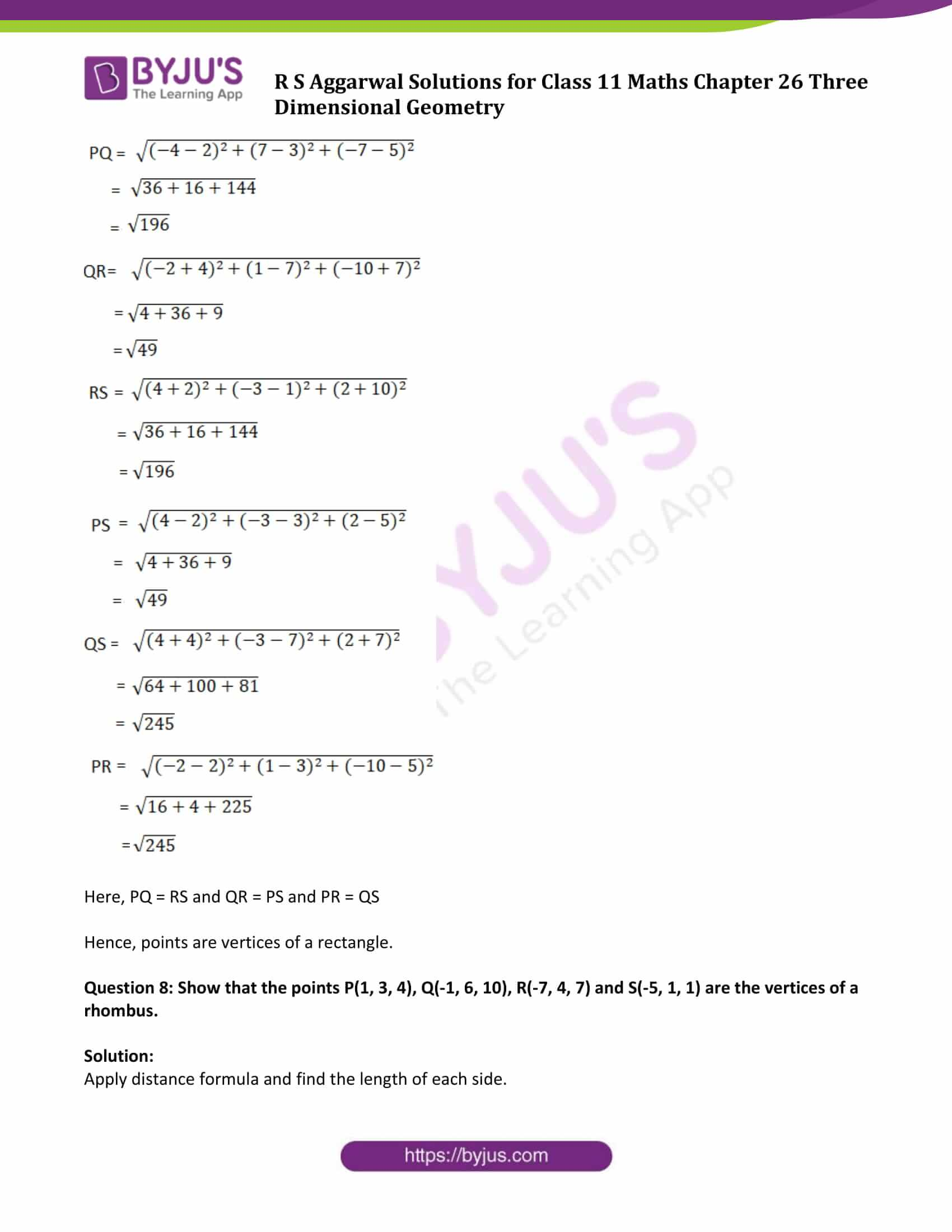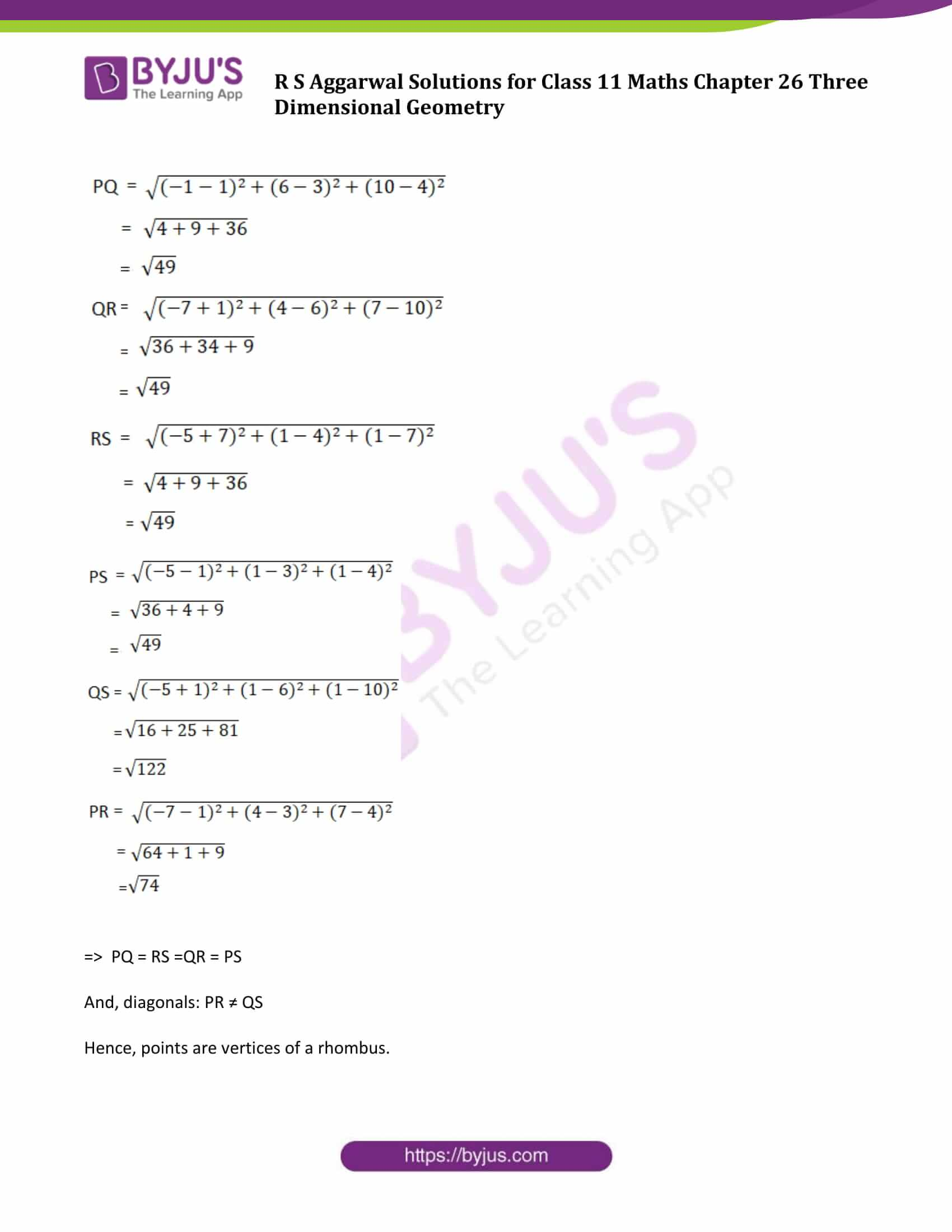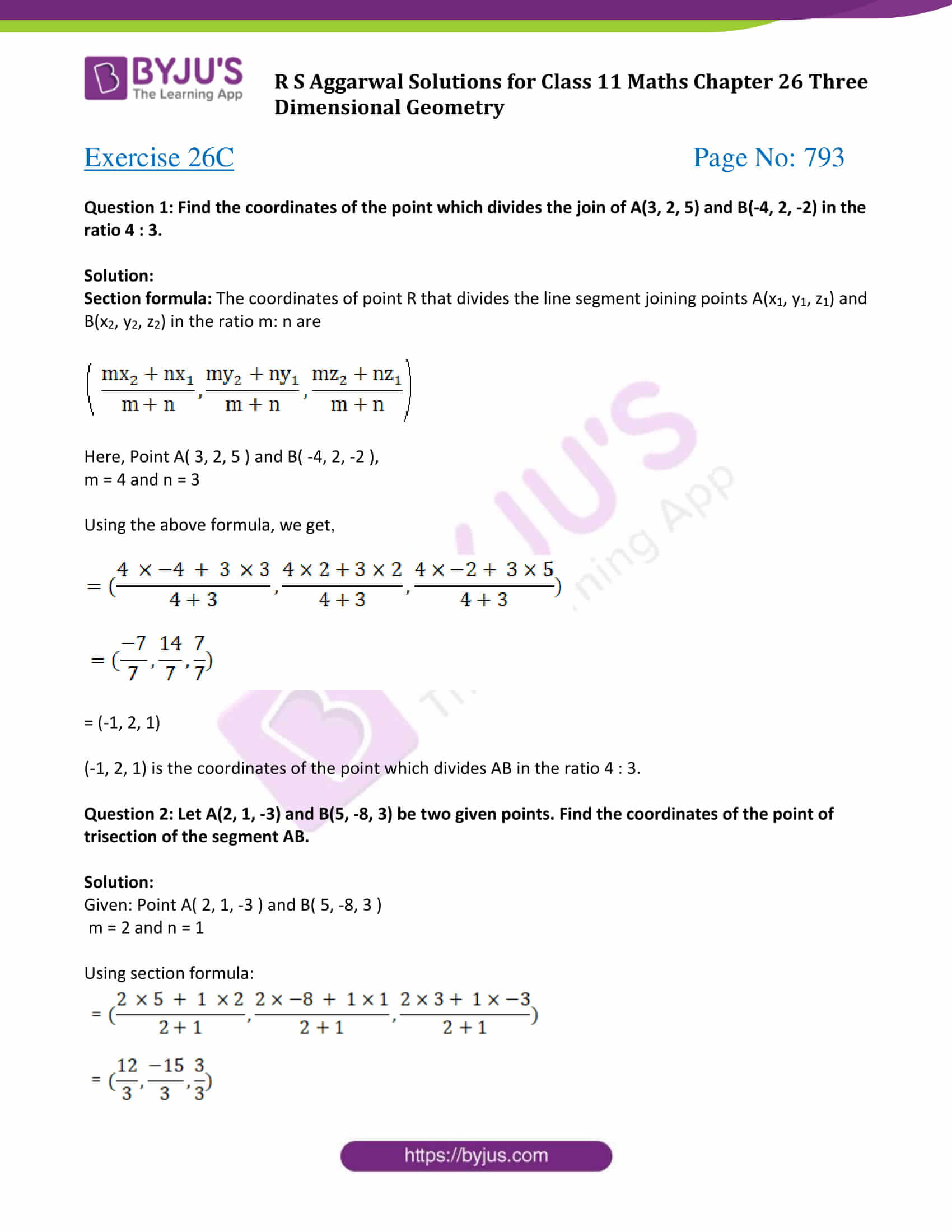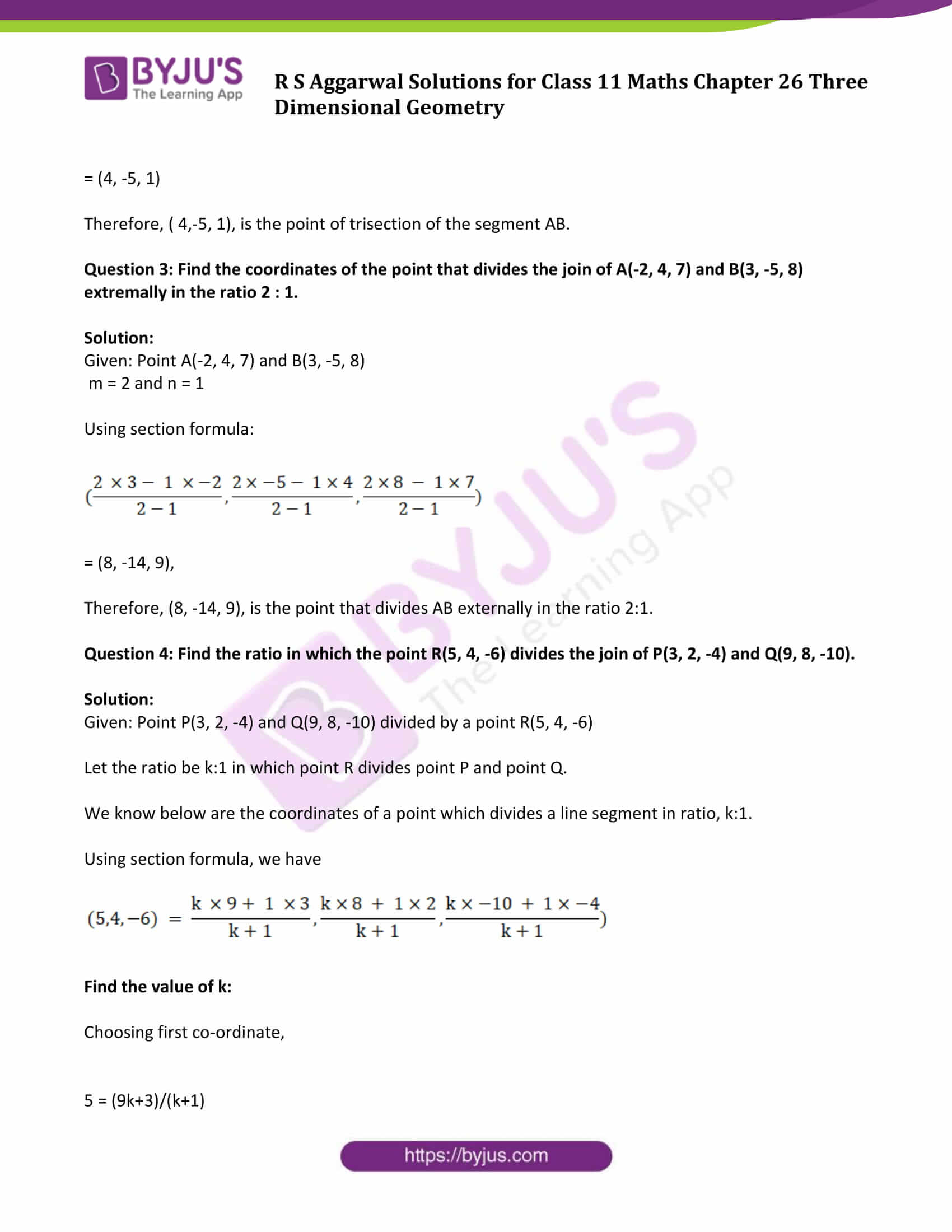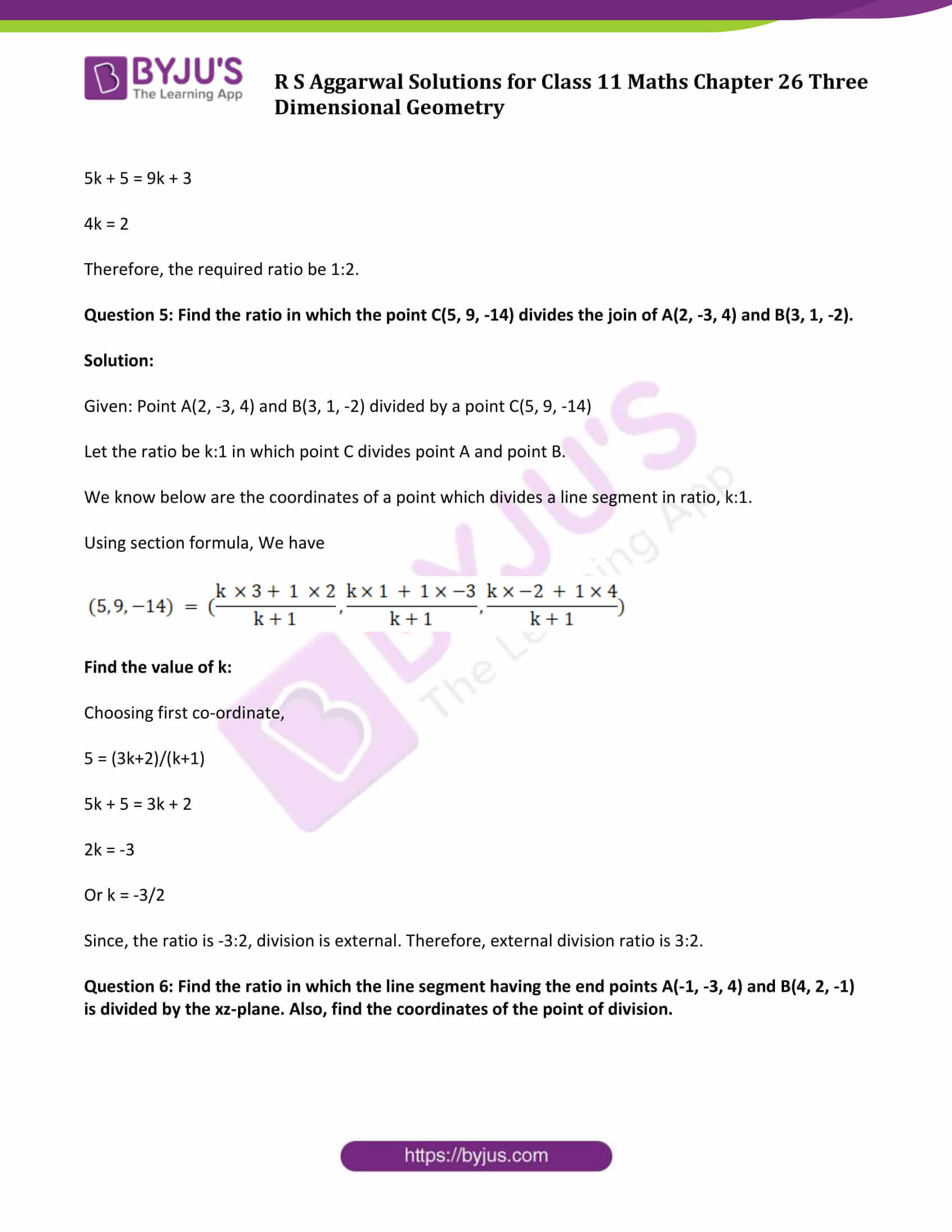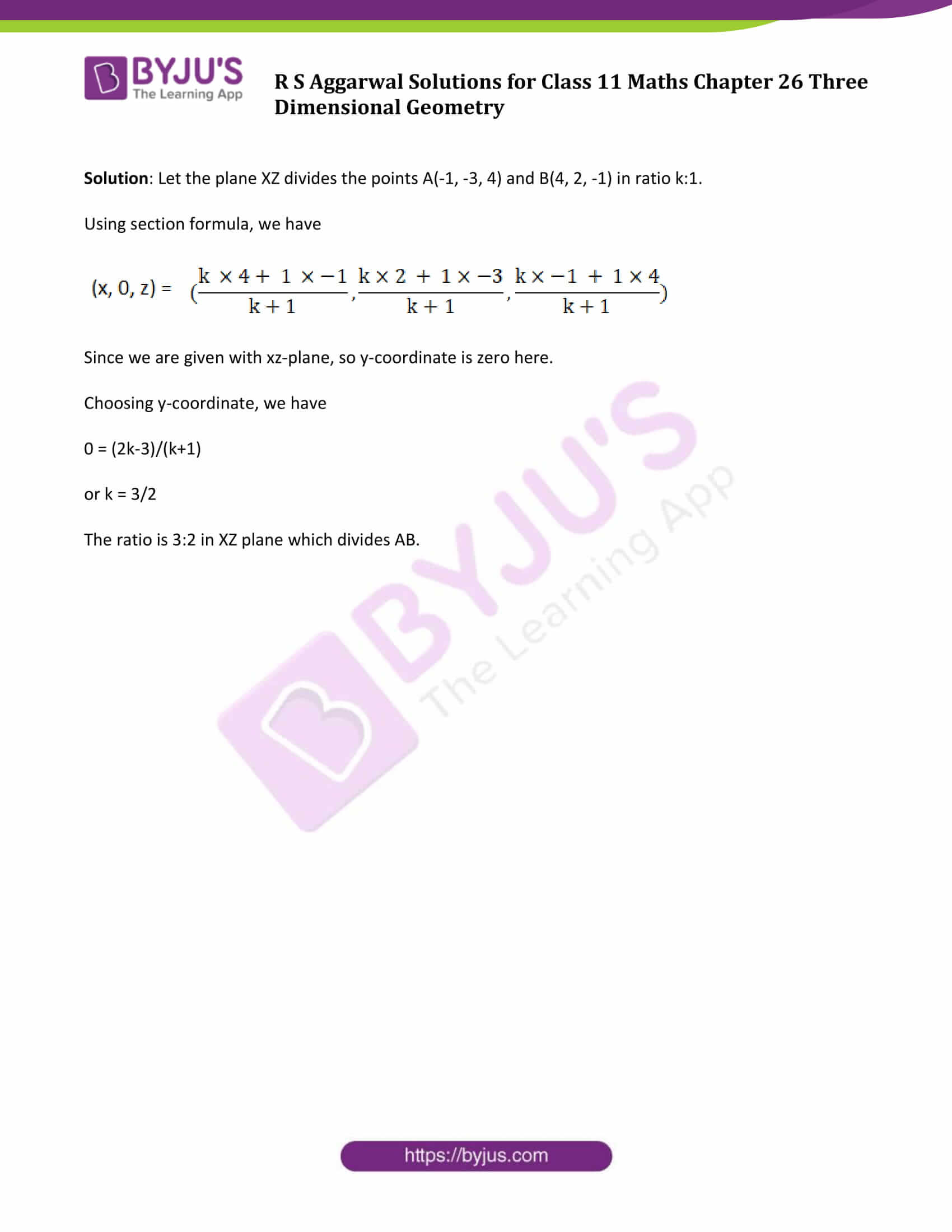### Access Answers of R S Aggarwal Solutions for Class 11 Maths Chapter 26 Three Dimensional Geometry

Exercise 26A Page No: 784

Question 1: If a point lies on the z-axis, then find its x-coordinate and y-coordinate.

Solution:

If the point lies on the z axis then its x-coordinate and y-coordinate will be zero.

Question 2: If a point lies on yz-plane then what is its x-coordinate?

Solution:

If the point lies on yz-plane then x-coordinate will be zero.

Question 3: In which plane does the point (4, -3, 0) lie?

Solution:

Given: x, y, z coordinates of the point are 4, -3, 0.

As the distance of point along the z-axis is 0, the plane in which the point lies is the xy-plane.

Question 4: In which octant does each of the given points lie?

(i) (-4, -1, -6)

(ii) (2, 3, -4)

(iii) (-6, 5, -1)

(iv) (4, -3, -2)

(v) (-1, -6, 5)

(vi) (4, 6, 8)

Solution:

The position of a point in a octant is signified by the signs of the x, y, z coordinates.

Signs of x, y, z coordinates in all the octants are as follow:

 Number Sign of X Sign of Y Sign of Z I + + + II – + + III – – + IV + – + V + + – VI – + – VII – – – VIII + – –

Compare signs with given table and allocate octants to the points

(i) (-4, -1, -6) lies in octant VII

(ii) (2, 3, -4) lies in octant V

(iii) (-6, 5, -1) lies in octant VI

(iv) (4, -3, -2) lies in octant VIII

(v) (-1, -6, 5) lies in octant III

(vi) (4, 6, 8) lies in octant I

Exercise 26B Page No: 787

Question 1: Find the distance between the points :

(i) A(5, 1, 2) and B(4, 6, -1)

(ii) P(1, -1, 3) and Q(2, 3, -5)

(iii) R(1, -3, 4) and S(4, -2, -3)

(iv) C(9, -12, -8) and the origin

Solution: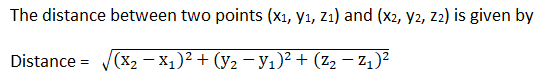(i) A(5, 1, 2) and B(4, 6, -1)

Here (x1, y1, z1) = (5, 1, 2) and (x2, y2, z2) = (4, 6, -1)

Now,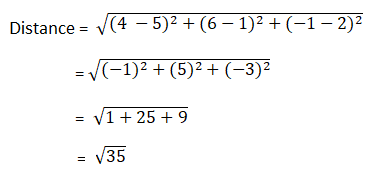Distance between A and B is √35 units

(ii) P(1, -1, 3) and Q(2, 3, -5)

Here (x1, y1, z1) = (1, -1, 3) and (x2, y2, z2) = (2, 3, -5)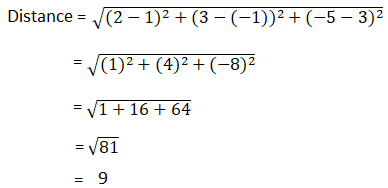Distance between P and Q is 9 units

(iii) R(1, -3, 4) and S(4, -2, -3)

Here (x1, y1, z1) = (1, -3, 4) and (x2, y2, z2) = (4, -2, -3)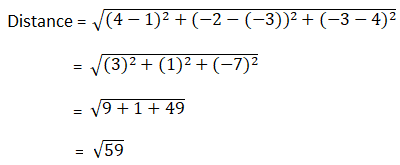Distance between R and S is √59 units

(iv) C(9, -12, -8) and the origin

Here (x1, y1, z1) = (9, -12, -8) and (x2, y2, z2) = (0, 0, 0)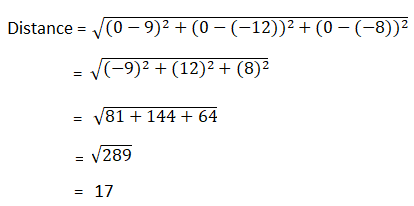Distance between C and Origin is 17 units.

Question 2: Show that the points A(1, -1, -5), B(3, 1,3) and C(9, 1, -3) are the vertices of an equilateral triangle.

Solution:

Given points are the vertices of an equilateral triangle if measure of all the sides are equal.

Apply distance formula:Length AB: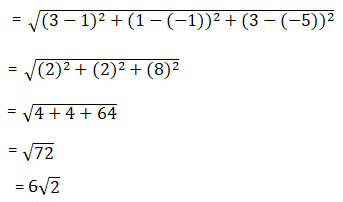Length BC: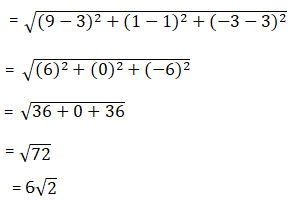Length AC: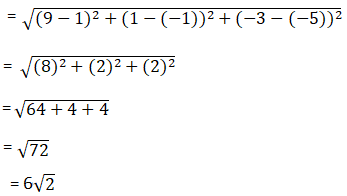=> AB = BC = AC

Therefore, Points A, B, C are vertices of an equilateral triangle.

Question 3: Show that the points A(4, 6, -5), B(0, 2, 3) and C(-4, -4, -1) from the vertices of an isosceles triangle.

Solution:

Given points are the vertices of an isosceles triangle if measure of any two sides are equal.

Apply distance formula:Length AB: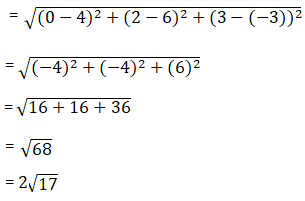Length BC: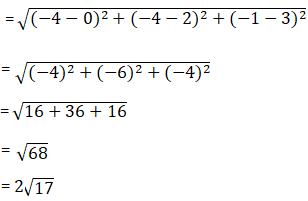Length AC: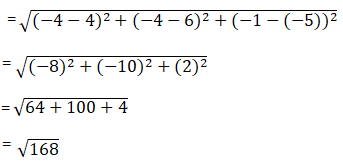From above result, we have AB = BC

Therefore, vertices A, B, C forms an isosceles triangle.

Question 4: Show that the points A(0, 1, 2), B(2, -1, 3) and C(1, -3, 1) are the vertices of an isosceles right-angled triangle.

Solution:

Given points are the vertices of an isosceles right-angled triangle if sum of squares of two sides is equal to square of third side.

Apply distance formula: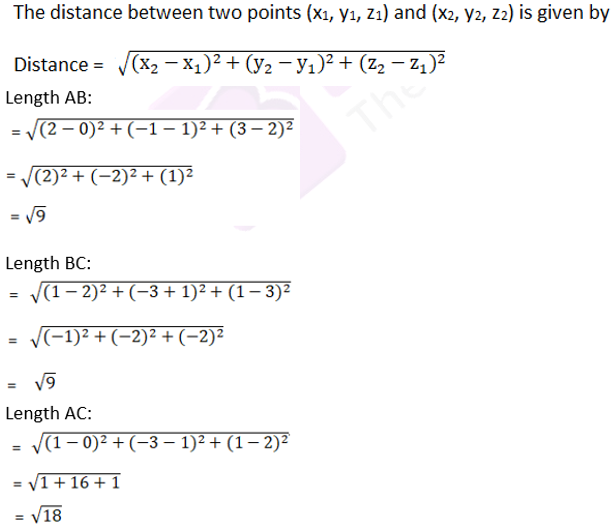Now, AB2 + BC2 = 9 + 9

= 18

= AC2

Therefore, Therefore, vertices A, B, C are the vertices of an isosceles right-angled triangle.

Question 5: Show that the points A(1, 1, 1), B(-2, 4, 1), C(1, -5, 5) and D(2, 2, 5) are the vertices of a square.

Solution:

Points A(1, 1, 1), B(-2, 4, 1), C(1, -5, 5) and D(2, 2, 5) are the vertices of a square if all sides are of equal measure.

Apply distance formula, and find the distance between all the points: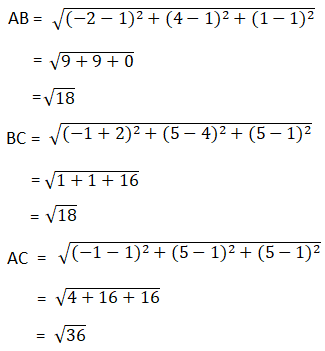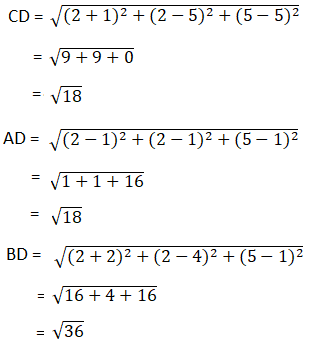From above results, AB = BC = CD = AD, and AC = BD

Hence vertices A, B, C, D form a square.

Question 6: Show that the points A(1, 2, 3), B(-1, -2, -1), C(2, 3, 2) and D(4, 7, 6) are the vertices of a parallelogram. Show that ABCD is not a rectangle.

Solution:

Apply distance formula, and find the distance between all the points: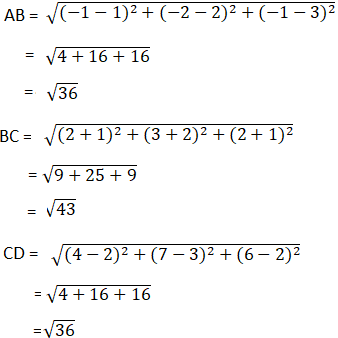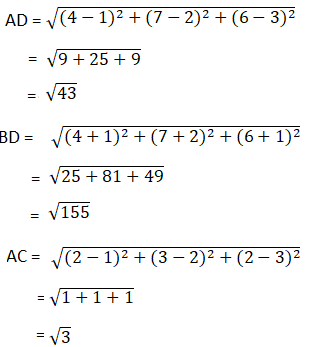From above result, we have

AB = CD [opposite sides]

Diagonal AC ≠ BD, Diagonals are not equal

Hence, Points are vertices of parallelogram and ABCD is not a rectangle.

Question 7: Show that the points P(2, 3, 5), Q(-4, 7, -7), R(-2, 1, -10) and S(4, -3, 2) are the vertices of a rectangle.

Solution:

PQRS is a rectangle if opposite sides are equal and diagonals are also of equal length.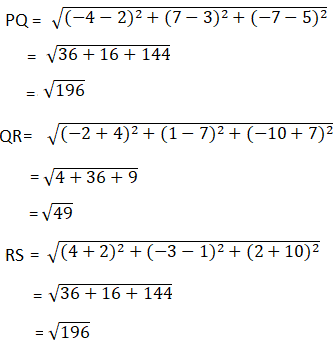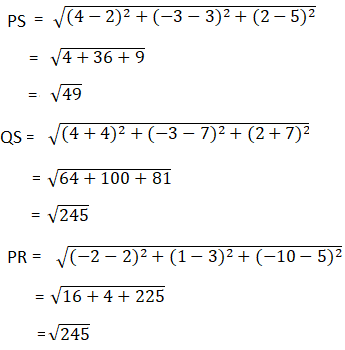Here, PQ = RS and QR = PS and PR = QS

Hence, points are vertices of a rectangle.

Question 8: Show that the points P(1, 3, 4), Q(-1, 6, 10), R(-7, 4, 7) and S(-5, 1, 1) are the vertices of a rhombus.

Solution:

Apply distance formula and find the length of each side.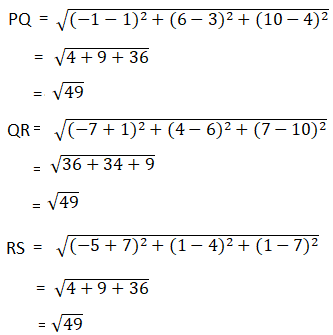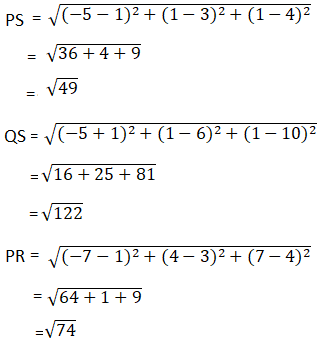=> PQ = RS =QR = PS

And, diagonals: PR ≠ QS

Hence, points are vertices of a rhombus.

Exercise 26C Page No: 793

Question 1: Find the coordinates of the point which divides the join of A(3, 2, 5) and B(-4, 2, -2) in the ratio 4 : 3.

Solution:

Section formula: The coordinates of point R that divides the line segment joining points A(x1, y1, z1) and B(x2, y2, z2) in the ratio m: n are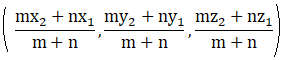Here, Point A( 3, 2, 5 ) and B( -4, 2, -2 ),

m = 4 and n = 3

Using the above formula, we get,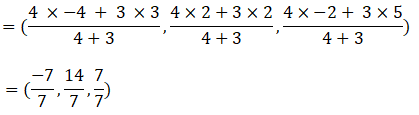= (-1, 2, 1)

(-1, 2, 1) is the coordinates of the point which divides AB in the ratio 4 : 3.

Question 2: Let A(2, 1, -3) and B(5, -8, 3) be two given points. Find the coordinates of the point of trisection of the segment AB.

Solution:

Given: Point A( 2, 1, -3 ) and B( 5, -8, 3 )

m = 2 and n = 1

Using section formula: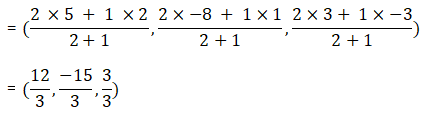= (4, -5, 1)

Therefore, ( 4,-5, 1), is the point of trisection of the segment AB.

Question 3: Find the coordinates of the point that divides the join of A(-2, 4, 7) and B(3, -5, 8) extremally in the ratio 2 : 1.

Solution:

Given: Point A(-2, 4, 7) and B(3, -5, 8)

m = 2 and n = 1

Using section formula: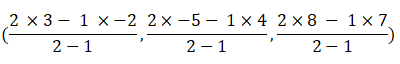= (8, -14, 9),

Therefore, (8, -14, 9), is the point that divides AB externally in the ratio 2:1.

Question 4: Find the ratio in which the point R(5, 4, -6) divides the join of P(3, 2, -4) and Q(9, 8, -10).

Solution:

Given: Point P(3, 2, -4) and Q(9, 8, -10) divided by a point R(5, 4, -6)

Let the ratio be k:1 in which point R divides point P and point Q.

We know below are the coordinates of a point which divides a line segment in ratio, k:1.

Using section formula, we have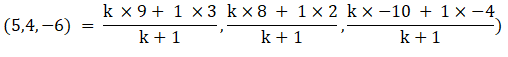Find the value of k:

Choosing first co-ordinate,

5 = (9k+3)/(k+1)

5k + 5 = 9k + 3

4k = 2

Therefore, the required ratio be 1:2.

Question 5: Find the ratio in which the point C(5, 9, -14) divides the join of A(2, -3, 4) and B(3, 1, -2).

Solution:

Given: Point A(2, -3, 4) and B(3, 1, -2) divided by a point C(5, 9, -14)

Let the ratio be k:1 in which point C divides point A and point B.

We know below are the coordinates of a point which divides a line segment in ratio, k:1.

Using section formula, We have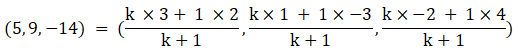Find the value of k:

Choosing first co-ordinate,

5 = (3k+2)/(k+1)

5k + 5 = 3k + 2

2k = -3

Or k = -3/2

Since, the ratio is -3:2, division is external. Therefore, external division ratio is 3:2.

Question 6: Find the ratio in which the line segment having the end points A(-1, -3, 4) and B(4, 2, -1) is divided by the xz-plane. Also, find the coordinates of the point of division.

Solution: Let the plane XZ divides the points A(-1, -3, 4) and B(4, 2, -1) in ratio k:1.

Using section formula, we have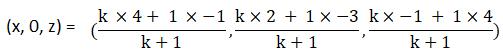Since we are given with xz-plane, so y-coordinate is zero here.

Choosing y-coordinate, we have

0 = (2k-3)/(k+1)

or k = 3/2

The ratio is 3:2 in XZ plane which divides AB.

R S Aggarwal Solutions For Class 11 Maths Chapter 26 Exercises:

Get detailed solutions for all the questions listed under below exercises:

Exercise 26A Solutions

Exercise 26B Solutions

Exercise 26C Solutions

## R S Aggarwal Solutions for Chapter 26 Three Dimensional Geometry

R S Aggarwal Chapter 26 – Three Dimensional Geometry, contains the answers to all the questions listed in this chapter.

Let us have a look at some of the topics that are being covered here:

• Three Dimensional Geometry Introduction
• Distance Formula
• Section formula
• Centroid of a triangle